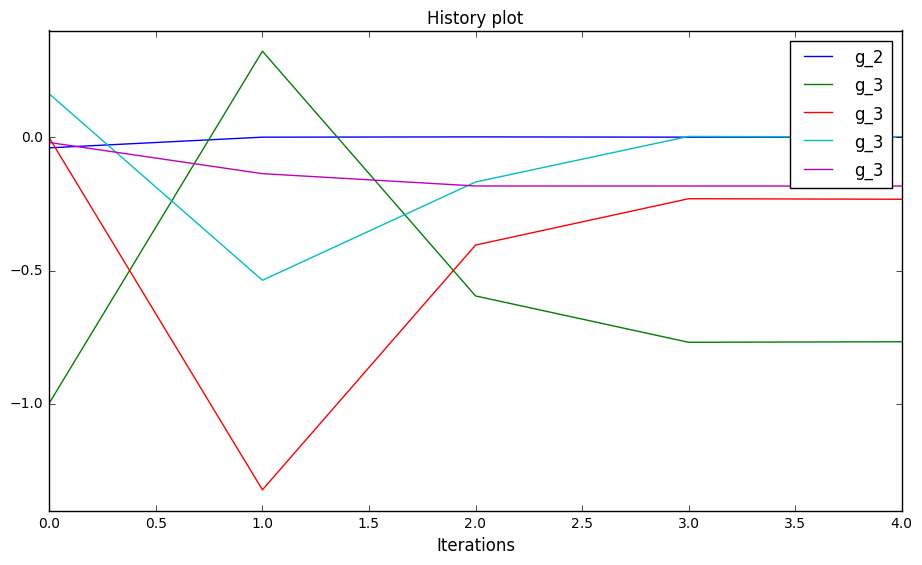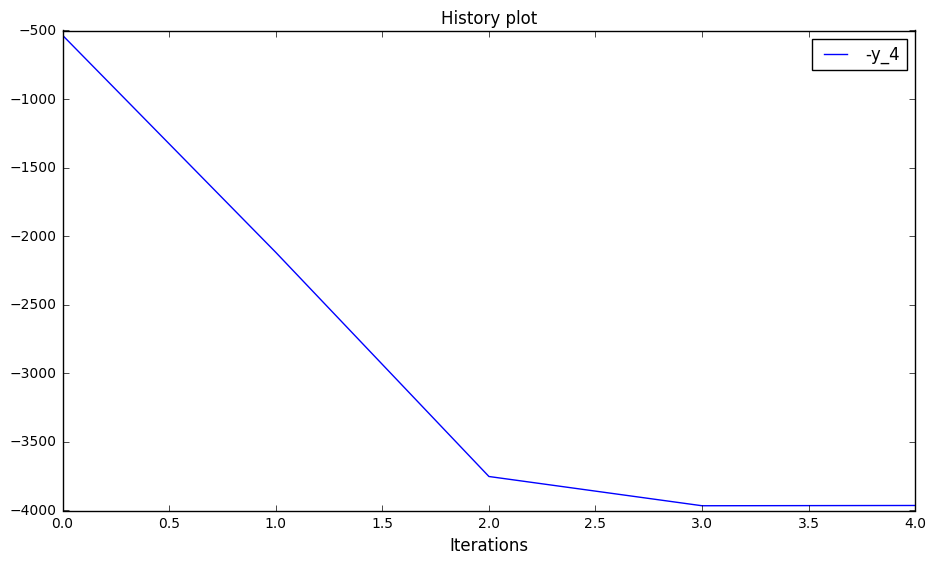# Basic history¶

## Preliminaries: instantiation and execution of the MDO scenario¶

Let’s start with the following code lines which instantiate and execute the MDOScenario :

from gemseo.api import create_discipline, create_scenario

formulation = 'MDF'

disciplines = create_discipline(["SobieskiPropulsion", "SobieskiAerodynamics",
"SobieskiMission", "SobieskiStructure"])

scenario = create_scenario(disciplines,
formulation=formulation,
objective_name="y_4",
maximize_objective=True,
design_space="design_space.txt")

scenario.set_differentiation_method("user")

algo_options = {'max_iter': 10, 'algo': "SLSQP"}
for constraint in ["g_1","g_2","g_3"]:

scenario.execute(algo_options)


## BasicHistory¶

### Description¶

The BasicHistory post-processing plots any of the constraint or objective functions w.r.t. optimization iterations or sampling snapshots.

The plot method requires the list of variable names to plot. It is possible either to save the plot, to show the plot or both.

### Options¶

• data_list, list(str) - list of variable names

• extension, str - file extension

• file_path, str - the base paths of the files to export

• save, bool - if True, exports plot to pdf

• show, bool - if True, displays the plot windows

## Case of the MDF formulation¶

Any of the functions stored in the optimization Database can be plotted using the basic history plot. For that, use the execute_post() API method with:

• the keyword "BasicHistory",

• the data_list to plot and

• additional arguments concerning the type of display (file, screen, both).

scenario.post_process("BasicHistory", save=True, show=False, file_path="basic_history",
data_list=["g_2", "g_3"])History of the constraints on the Sobieski use case for the MDF formulation using the basic history plot

Warning

In the Database, when the aim of the optimization problem is to maximize the objective function, the objective function name is preceded by a “-” and the stored values are the opposite of the objective function.

scenario.post_process("BasicHistory", save=True, show=False, file_path="basic_history",
data_list=["-y_4"])History of the opposite of the objective function on the Sobieski use case for the MDF formulation using the basic history plot